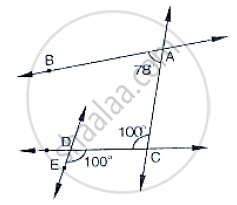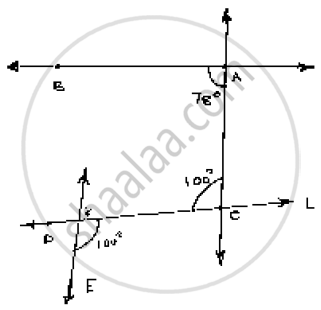# In the Below Fig, State Which Lines Are Parallel and Why? - Mathematics

In the below fig, state which lines are parallel and why?#### SolutionVertically opposite angles are equal

∠EOC = ∠DOK =100°

Angle ∠DOK = ∠ACO =100°

Here two lines EK and CA cut by a third line ‘l’ and the corresponding angles to it are

equal  ∴  EK || AC.

Is there an error in this question or solution?
Chapter 10: Lines and Angles - Exercise 10.4 [Page 46]

#### APPEARS IN

RD Sharma Mathematics for Class 9
Chapter 10 Lines and Angles
Exercise 10.4 | Q 4 | Page 46

Share